# Gas Laws Boyles Law Charless law GayLussacs Law

• Slides: 23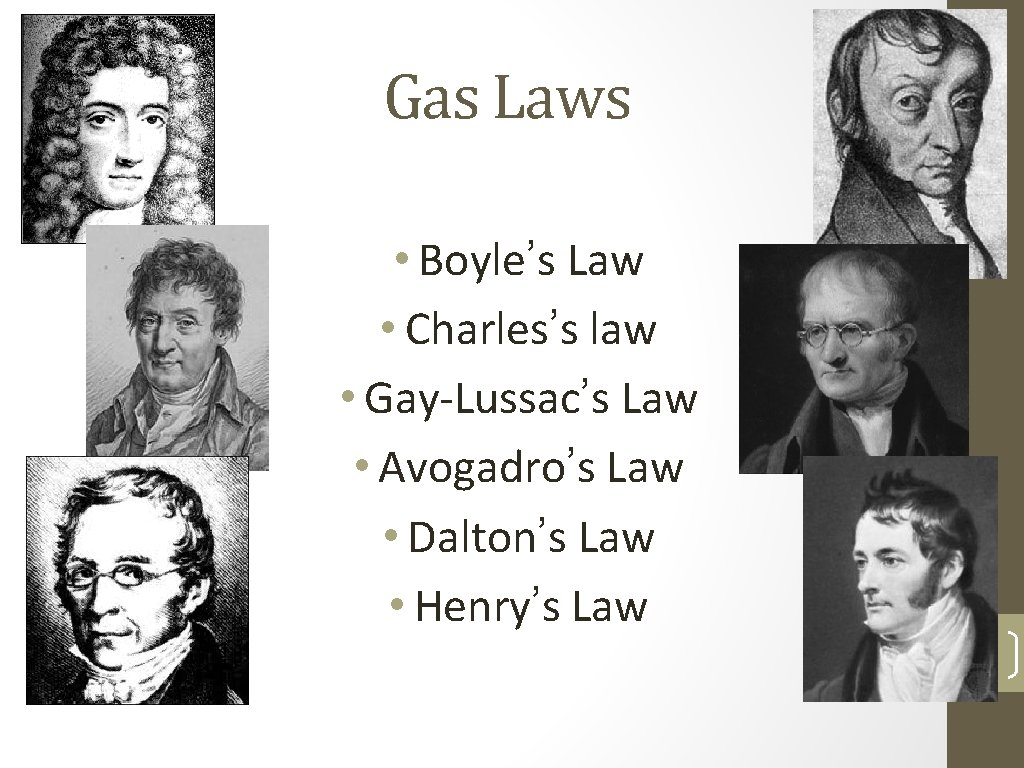Gas Laws • Boyle’s Law • Charles’s law • Gay-Lussac’s Law • Avogadro’s Law • Dalton’s Law • Henry’s Law 1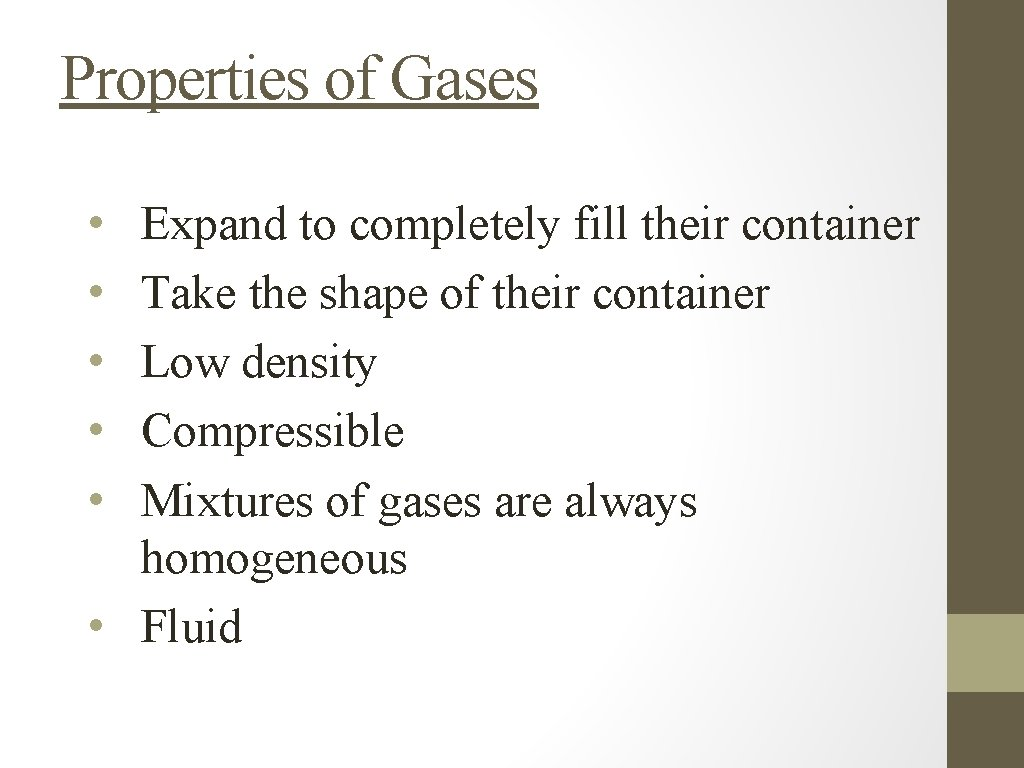Properties of Gases • • • Expand to completely fill their container Take the shape of their container Low density Compressible Mixtures of gases are always homogeneous • FluidLets talk units for Gas Laws P, V, n, T • We need four variables: • Volume (V) in Liters • Pressure (P) ~ next slide • Temperature (T) in Kelvin (T(K) = T(o. C) + 273) • Moles (n) in moles 3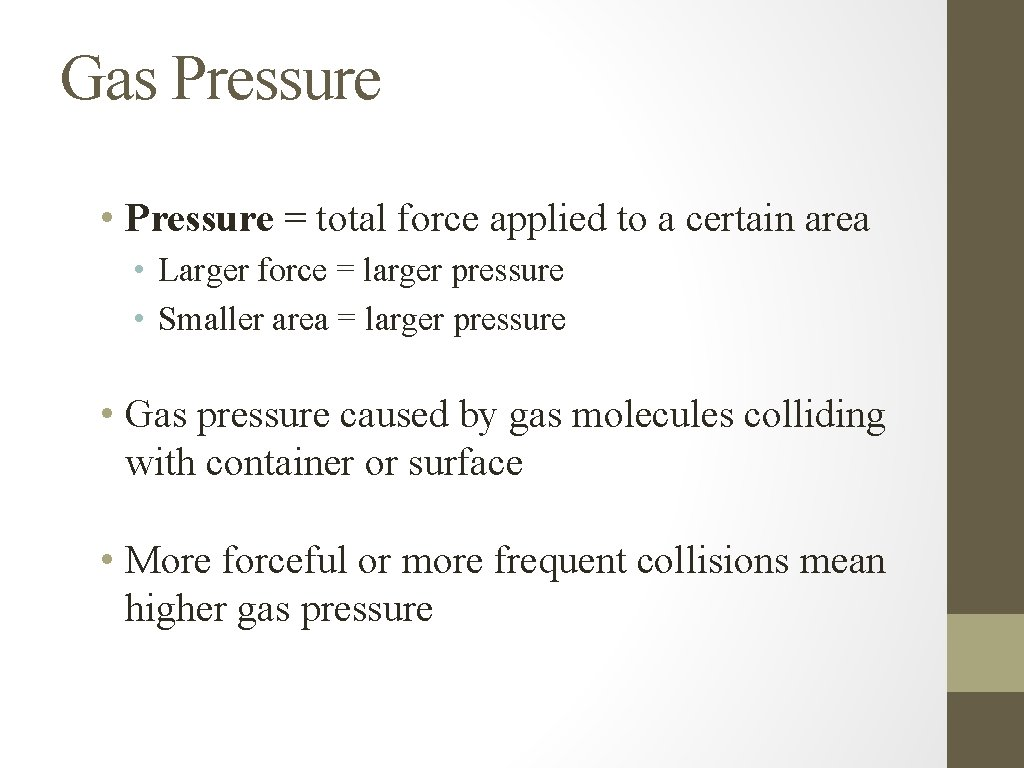Gas Pressure • Pressure = total force applied to a certain area • Larger force = larger pressure • Smaller area = larger pressure • Gas pressure caused by gas molecules colliding with container or surface • More forceful or more frequent collisions mean higher gas pressure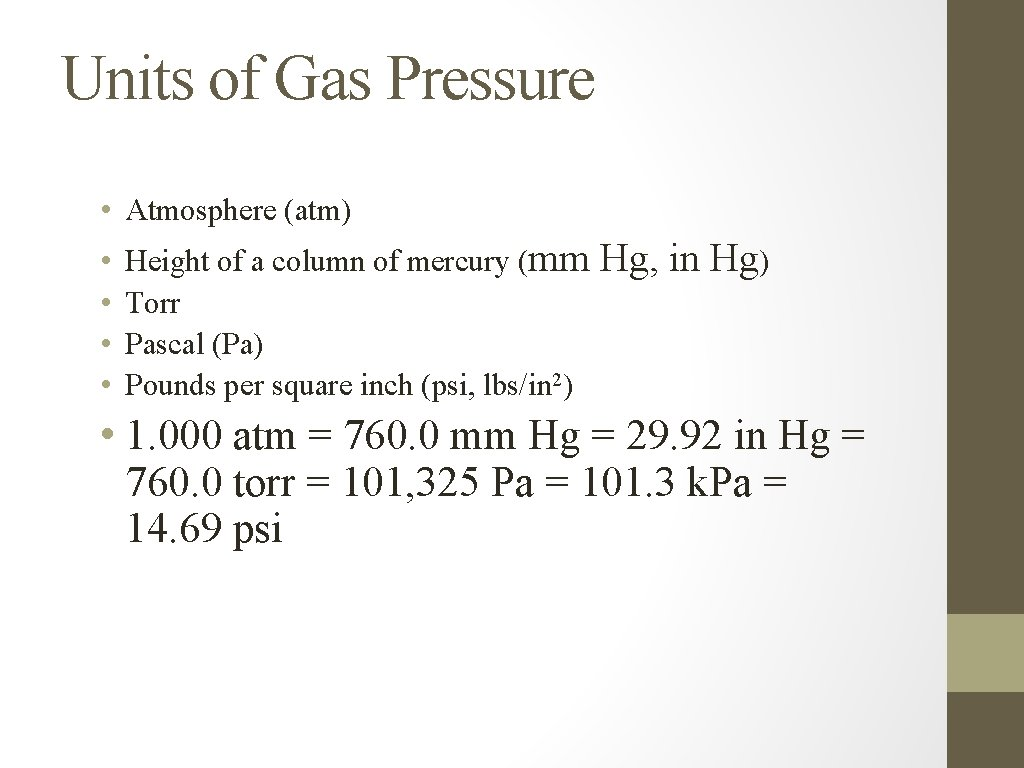Units of Gas Pressure • Atmosphere (atm) • • Height of a column of mercury (mm Torr Pascal (Pa) Pounds per square inch (psi, lbs/in 2) Hg, in Hg) • 1. 000 atm = 760. 0 mm Hg = 29. 92 in Hg = 760. 0 torr = 101, 325 Pa = 101. 3 k. Pa = 14. 69 psi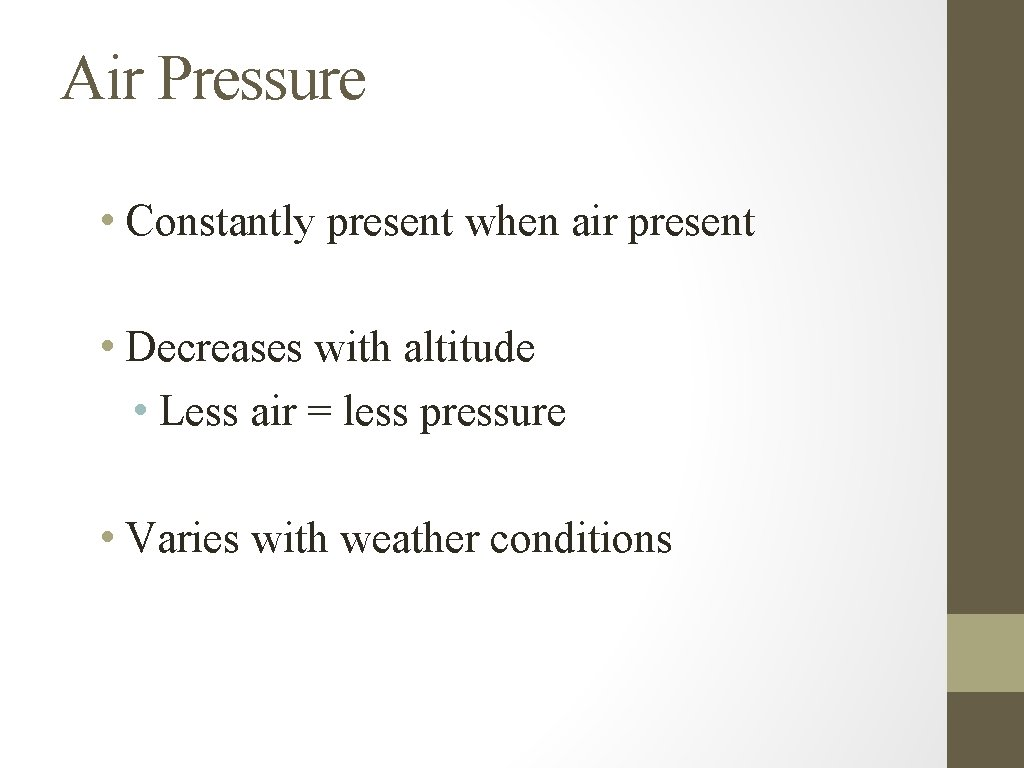Air Pressure • Constantly present when air present • Decreases with altitude • Less air = less pressure • Varies with weather conditions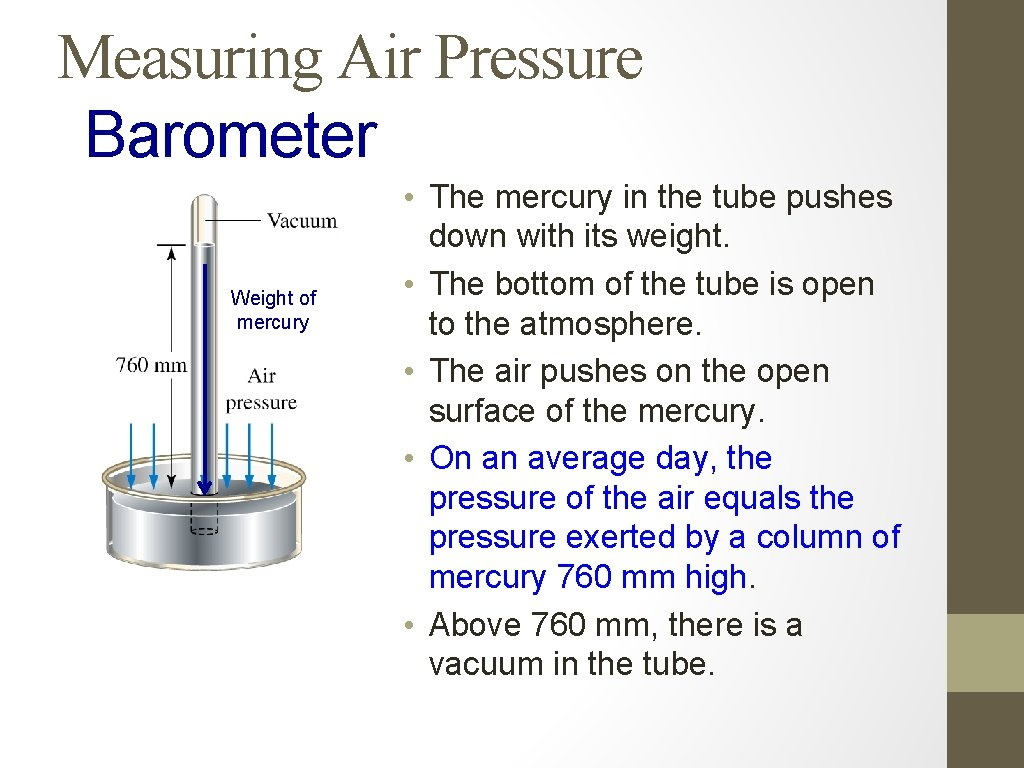Measuring Air Pressure Barometer Weight of mercury • The mercury in the tube pushes down with its weight. • The bottom of the tube is open to the atmosphere. • The air pushes on the open surface of the mercury. • On an average day, the pressure of the air equals the pressure exerted by a column of mercury 760 mm high. • Above 760 mm, there is a vacuum in the tube.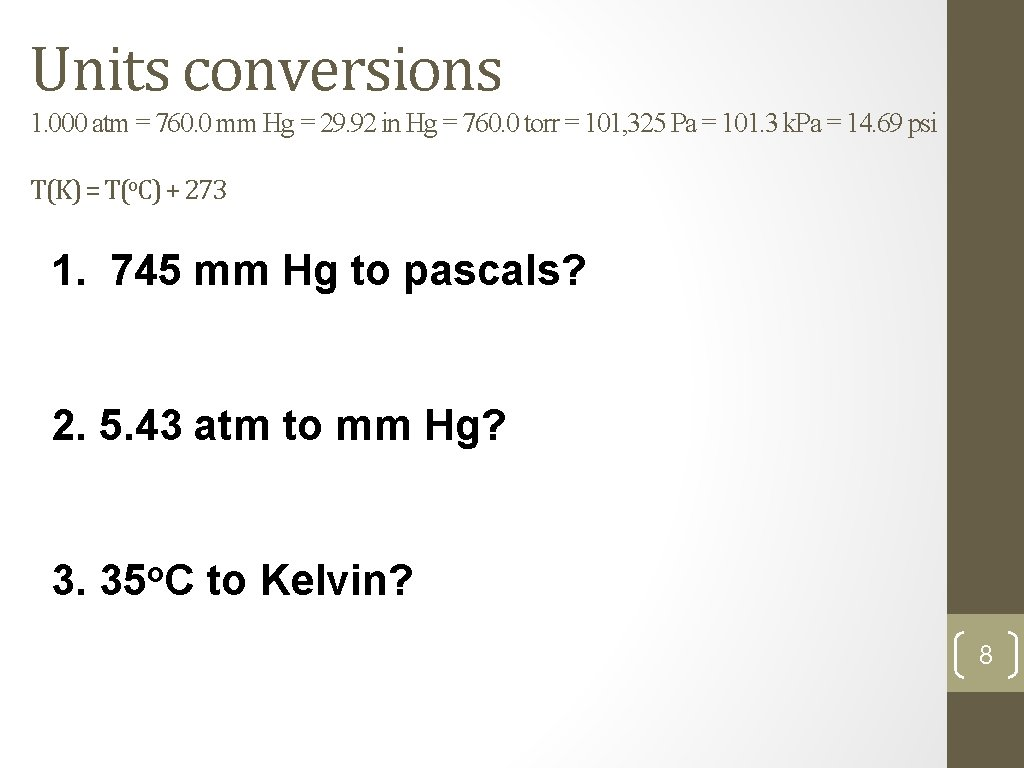Units conversions 1. 000 atm = 760. 0 mm Hg = 29. 92 in Hg = 760. 0 torr = 101, 325 Pa = 101. 3 k. Pa = 14. 69 psi T(K) = T(o. C) + 273 1. 745 mm Hg to pascals? 2. 5. 43 atm to mm Hg? 3. 35 o. C to Kelvin? 8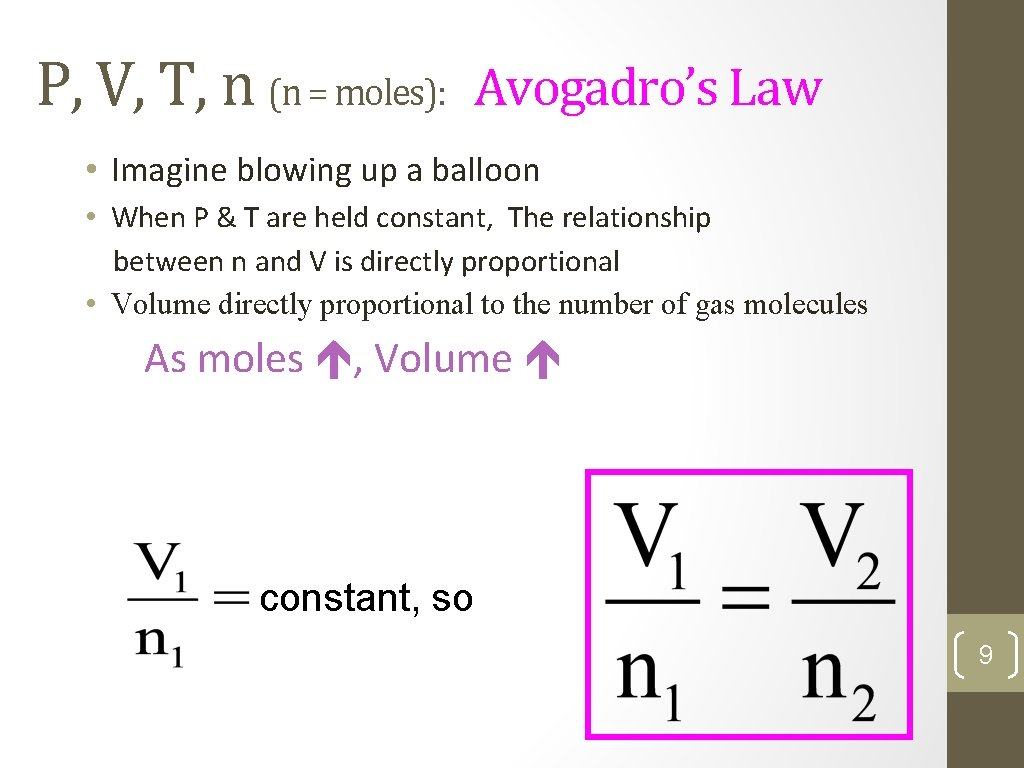P, V, T, n (n = moles): Avogadro’s Law • Imagine blowing up a balloon • When P & T are held constant, The relationship between n and V is directly proportional • Volume directly proportional to the number of gas molecules As moles , Volume constant, so 9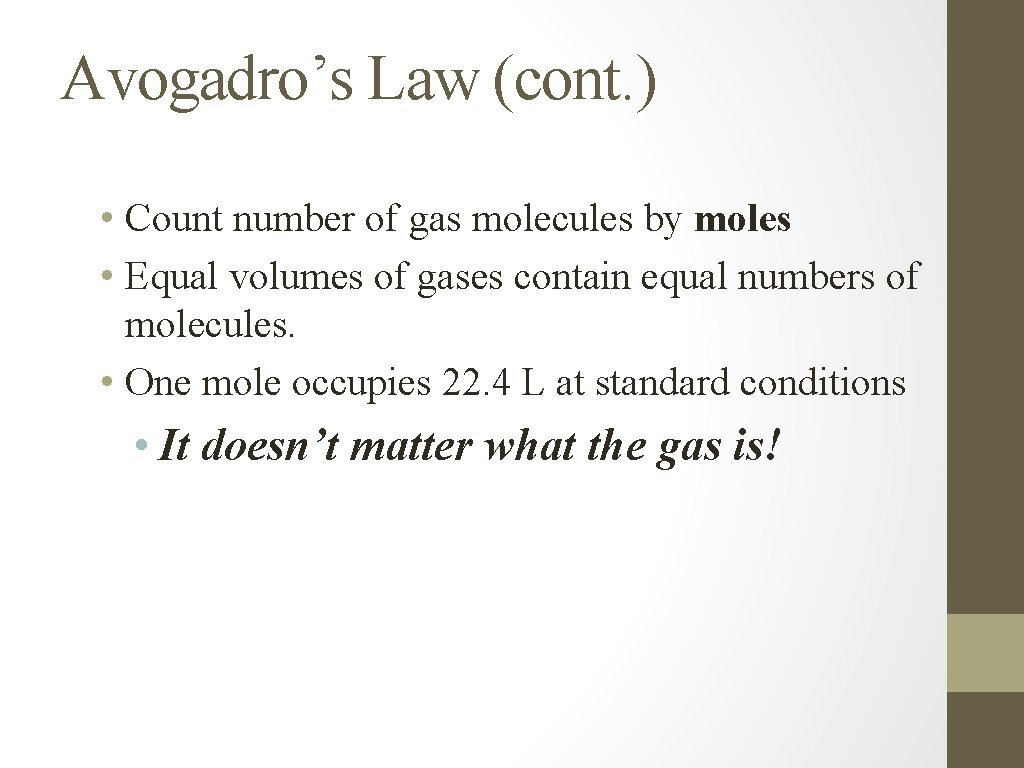Avogadro’s Law (cont. ) • Count number of gas molecules by moles • Equal volumes of gases contain equal numbers of molecules. • One mole occupies 22. 4 L at standard conditions • It doesn’t matter what the gas is!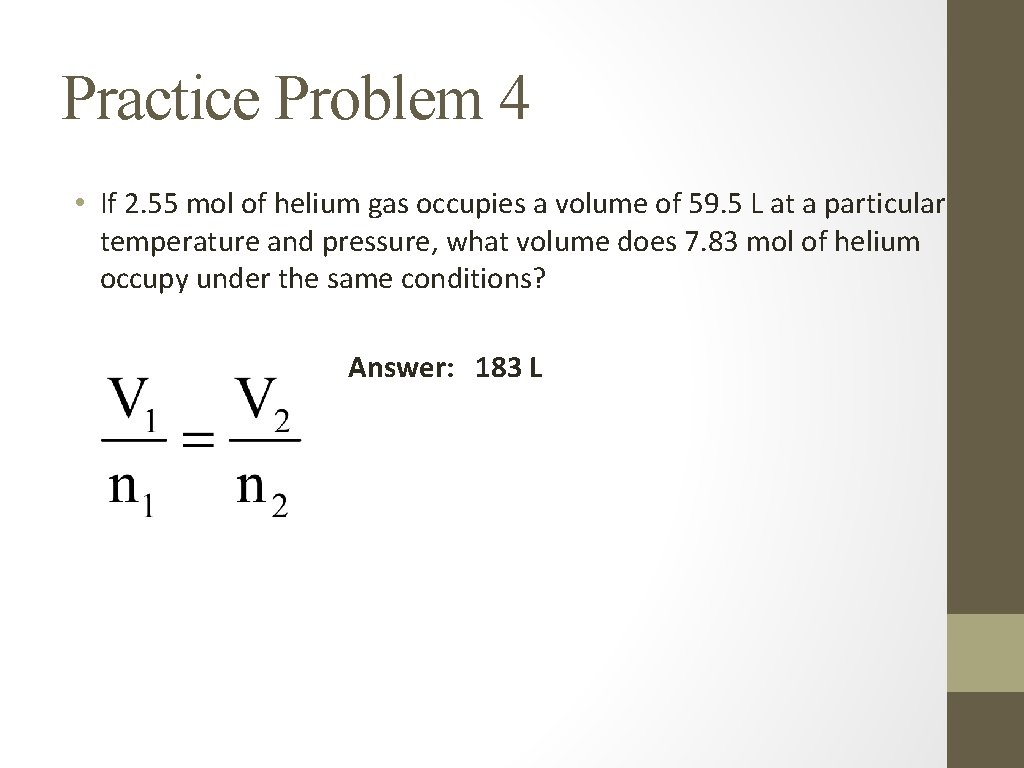Practice Problem 4 • If 2. 55 mol of helium gas occupies a volume of 59. 5 L at a particular temperature and pressure, what volume does 7. 83 mol of helium occupy under the same conditions? Answer: 183 LP, V, T, n (n = moles): Boyle’s Law • When n & T are held constant, the relationship between P and V is inversely proportional. As Pressure , Volume • P x V = constant, so P 1 x V 1 = P 2 x V 2 12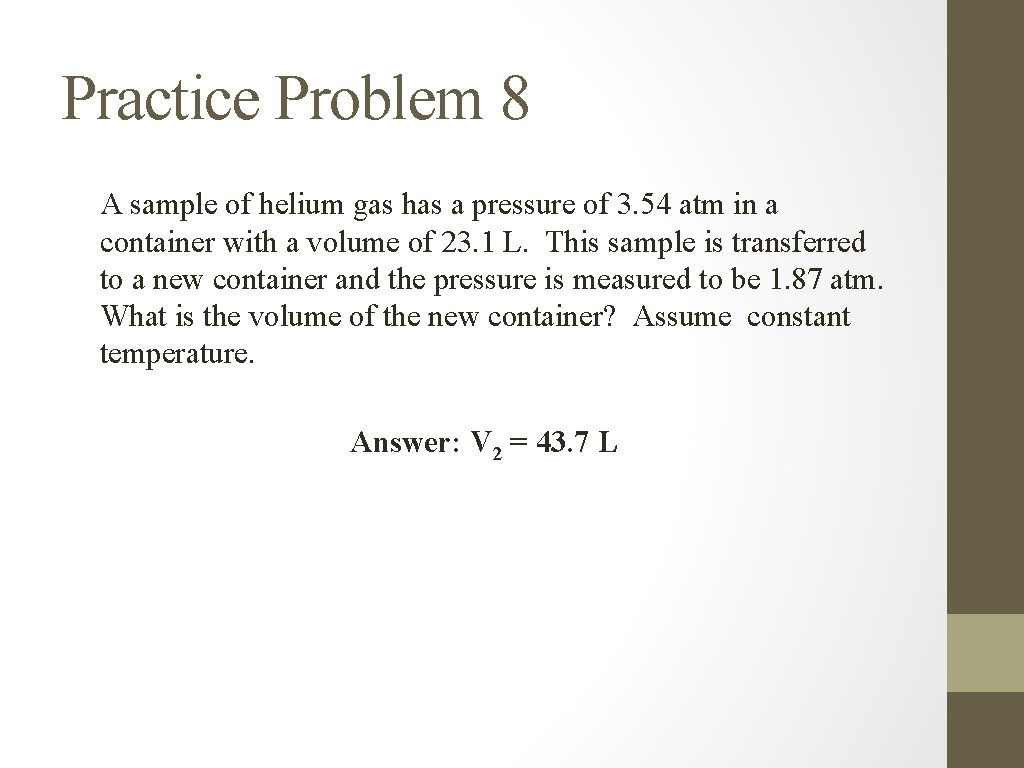Practice Problem 8 A sample of helium gas has a pressure of 3. 54 atm in a container with a volume of 23. 1 L. This sample is transferred to a new container and the pressure is measured to be 1. 87 atm. What is the volume of the new container? Assume constant temperature. Answer: V 2 = 43. 7 L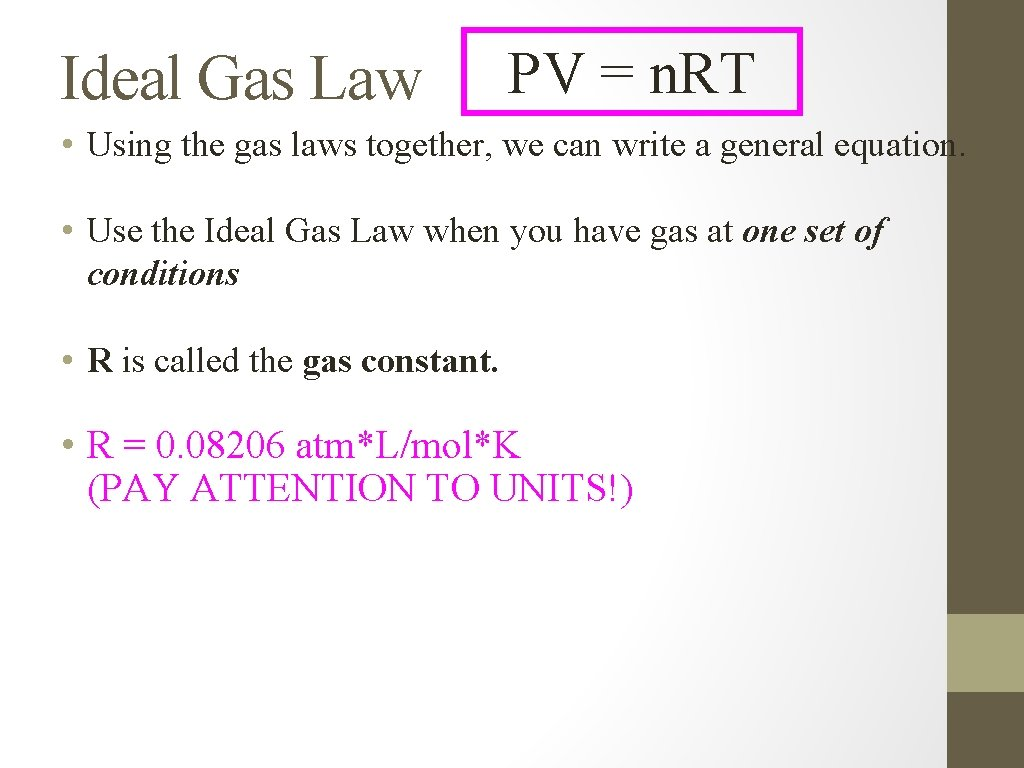Ideal Gas Law PV = n. RT • Using the gas laws together, we can write a general equation. • Use the Ideal Gas Law when you have gas at one set of conditions • R is called the gas constant. • R = 0. 08206 atm*L/mol*K (PAY ATTENTION TO UNITS!)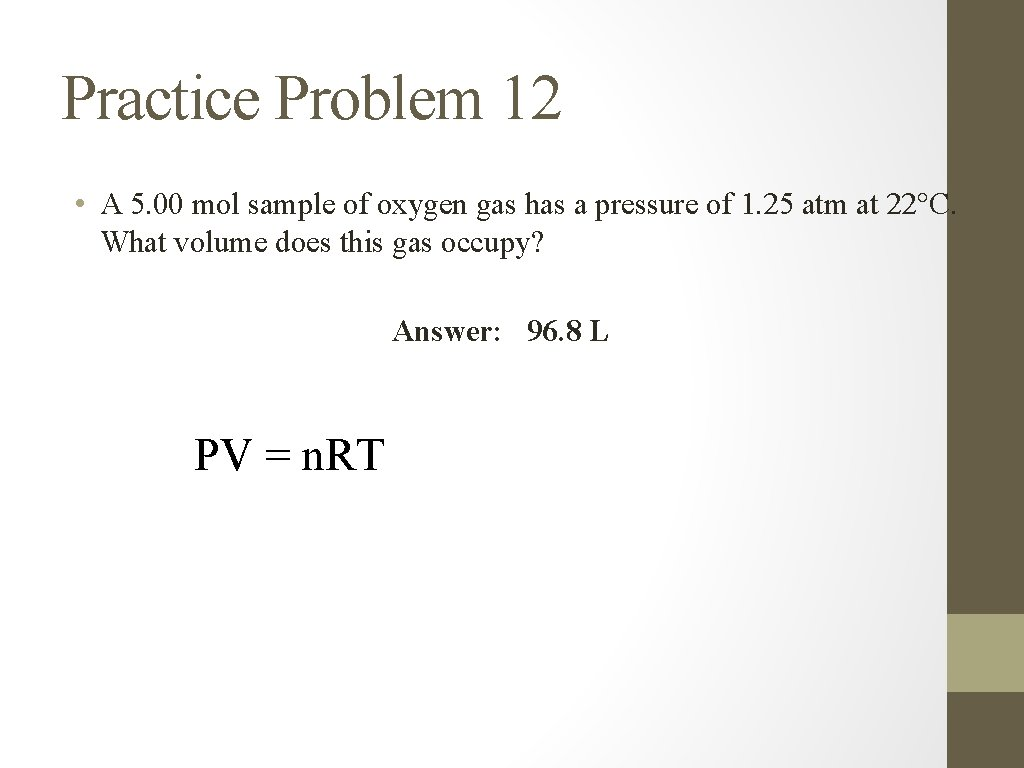Practice Problem 12 • A 5. 00 mol sample of oxygen gas has a pressure of 1. 25 atm at 22°C. What volume does this gas occupy? Answer: 96. 8 L PV = n. RT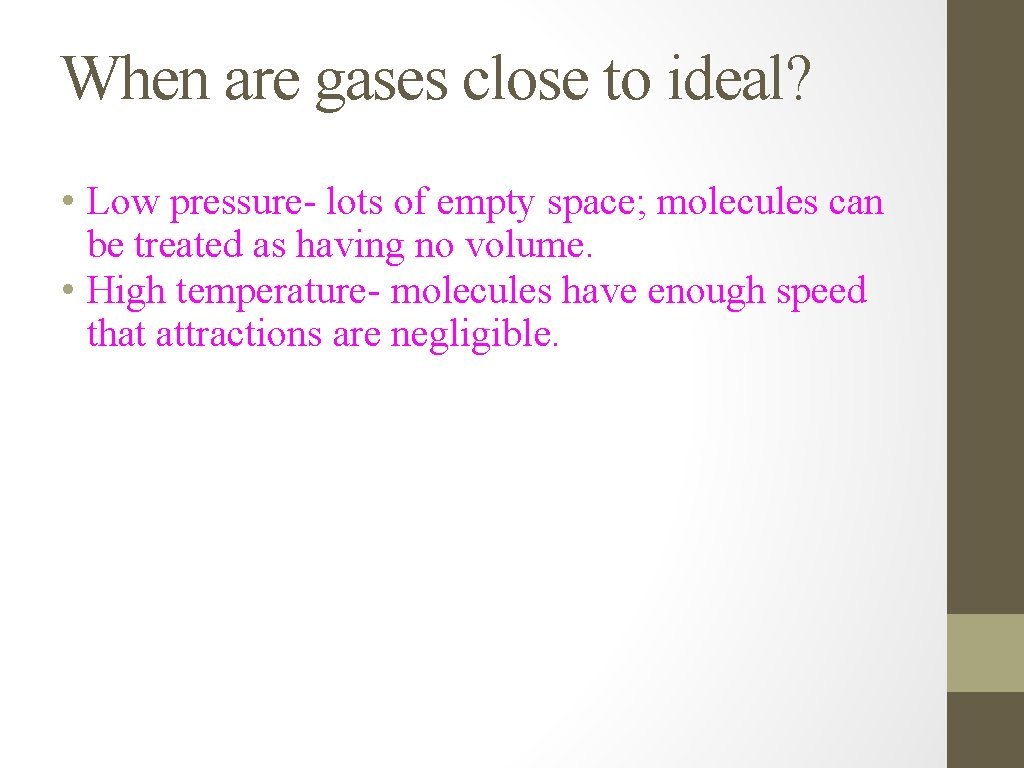When are gases close to ideal? • Low pressure- lots of empty space; molecules can be treated as having no volume. • High temperature- molecules have enough speed that attractions are negligible.Read Ch. 13 Do self-check problems Also do end-of-chapter problems: 7, 11, 18, 20, 32, 34, 42, 50, 52, 56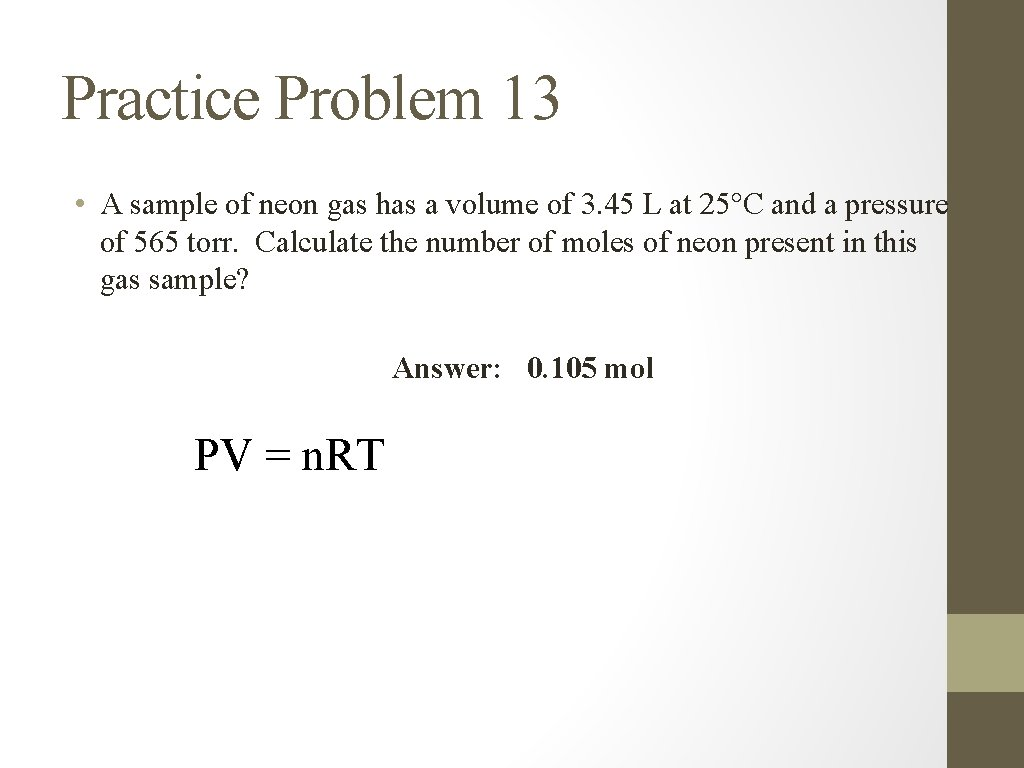Practice Problem 13 • A sample of neon gas has a volume of 3. 45 L at 25°C and a pressure of 565 torr. Calculate the number of moles of neon present in this gas sample? Answer: 0. 105 mol PV = n. RTPractice Problem 14 • A 0. 250 mol sample of argon gas has a volume of 9. 00 L at a pressure of 875 mm Hg. What is the temperature (in °C) of the gas? Answer: 232 °C PV = n. RT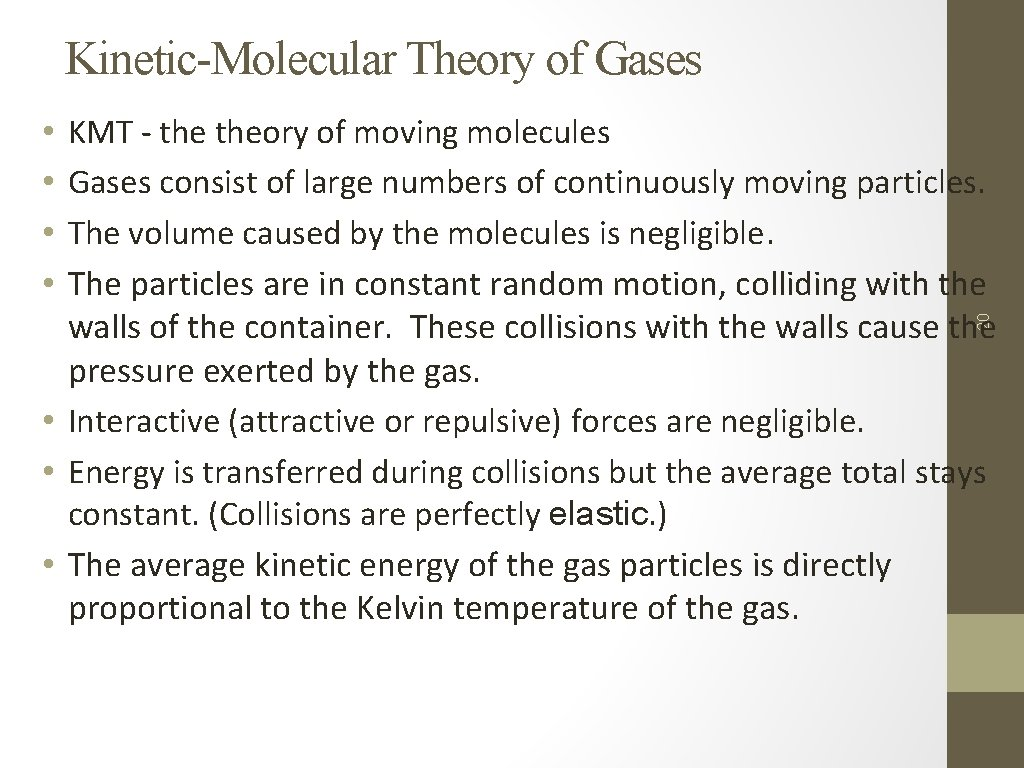Kinetic-Molecular Theory of Gases KMT - theory of moving molecules Gases consist of large numbers of continuously moving particles. The volume caused by the molecules is negligible. The particles are in constant random motion, colliding with the walls of the container. These collisions with the walls cause the pressure exerted by the gas. • Interactive (attractive or repulsive) forces are negligible. • Energy is transferred during collisions but the average total stays constant. (Collisions are perfectly elastic. ) • The average kinetic energy of the gas particles is directly proportional to the Kelvin temperature of the gas. 20 • •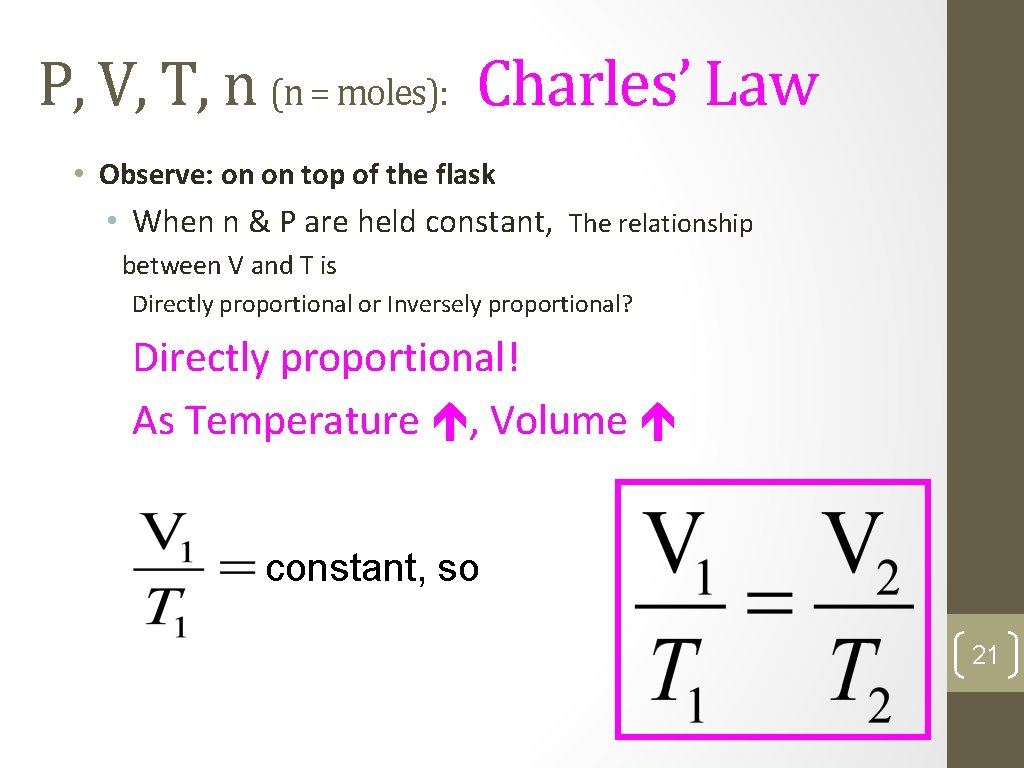P, V, T, n (n = moles): Charles’ Law • Observe: on on top of the flask • When n & P are held constant, The relationship between V and T is Directly proportional or Inversely proportional? Directly proportional! As Temperature , Volume constant, so 21Practice Problem 6 A sample of oxygen gas has a volume of 4. 55 L at 25°C. Calculate the volume of the oxygen gas when the temperature is raised to 45°C. Assume constant pressure • V 1 T 1 = V 2 T 2 Answer: V 2 = 4. 86 LP, V, T, n (n = moles): Gay-Lussac’s Law • Observe: Absolute Zero devise and Soda can • When n & V are held constant, The relationship between P and T is Directly proportional or Inversely proportional? Directly proportional! As Temperature , Pressure constant, so 23